## Divisibility of 2n choose n by a prime.

### Penelope Nom

A recent question and answer sent to Quandaries and Queries was

Your question is an unsolved problem.

Erdos, Graham, Rusza and Straus (Math. of Comp., 29(1975),pp 83-92) show that for any two primes p and q there exist infinitely many integers n for which (C(2n,n),pq) = 1. They remark that nothing is known for three primes and, in particular, they ask whether there are infinitely many n for which (C(2n,n),105) = 1 and this is your problem. As far as I know this is still unsettled.

If we want to look at prime factors of n! there is a nice way to find the power to which a prime p occurs dating back to Legendre. Legendre observed that if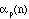is the power to which p divides n! then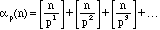where the square brackets [] denote the greatest integer function. For example, the largest power of 3 in 204! is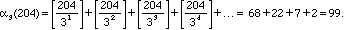This is because 3 divides every 3rd number in the sequence 1, 2, 3, ... , 204; 32 divides every 9th number in the sequence 1, 2, 3 , ... , 204; and 33 divides every 27th number in the sequence 1, 2, 3, ... , 204; and 34 divides every 81st number in the sequence 1, 2, 3, ... , 204.

Similarly if you wanted to find out how many zeroes appear at the end of 204! what you really need to find out is how often 5 divides 204! That's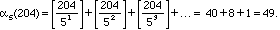Note that if we write 204 in base 3 (see Converting to other bases in Quandaries & Queries), i.e. 204 =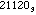the sum of the digits in base 3 is 6. If we write 204 in base 5 we get 204 =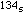the sum of the digits is 8. Observe that (204 - 6 )/(3 - 1) = 99. Observe also that

(204 - 8 )/(5 - 1) = 49. Is this an accident, getting 99 and 49 again this way? It isn't. The proof is a little messy but not too hard, however we won't go through it here. Let's have a peek at what goes on though.

Let me write Sp(n) for the sum of the digits of n in base p. We can in general show that the power to which p divides n! can be expressed as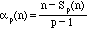. The interested reader might then want to see how often a prime p divides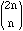, as was asked in the Quandaries and Queries question, call it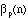; we find, looking at the factorials in the numerator and denominator ofthat=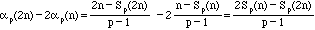.

For example, 204 =and 102 =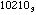. Thus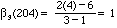. Similarly 204 =, 102 =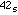thus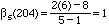. That is both 3 and 5 divide 204 choose 102 to the first power only.

Go to Math Central